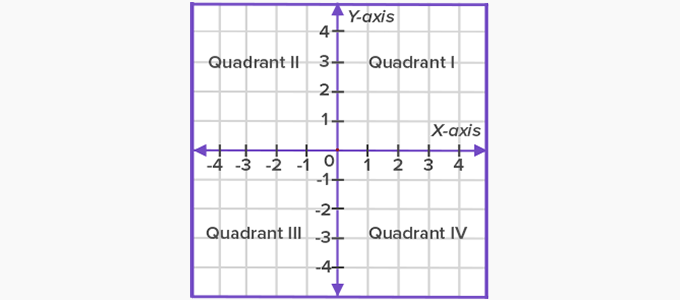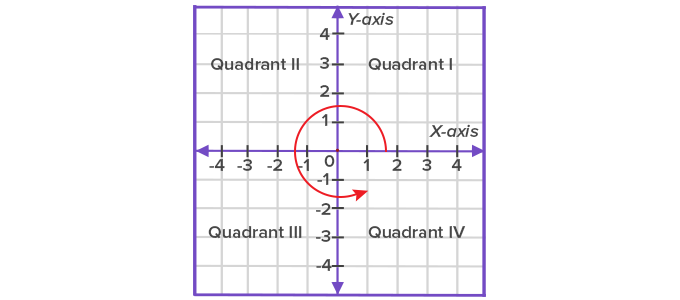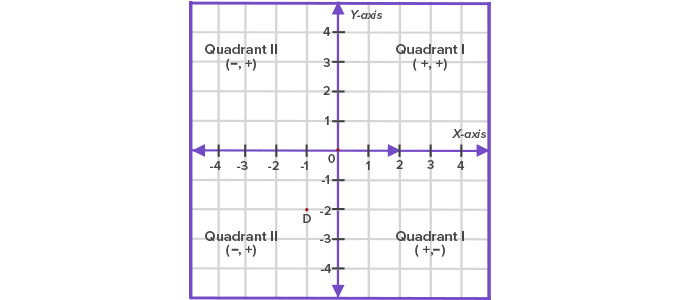# Quadrant - Definition with Examples

The Complete K-5 Math Learning Program Built for Your Child

• 30 Million Kids

Loved by kids and parent worldwide

• 50,000 Schools

Trusted by teachers across schools

• Comprehensive Curriculum

Aligned to Common Core

Take a sheet of paper and draw the horizontal axis (x-axis) and the vertical axis (y-axis). These two axes divide the paper into 4 parts. Each part is called a quadrant.The meeting point of the two axes is called the origin.By convention, quadrants are named in an anticlockwise direction.

Each point is represented by its x-coordinate followed by its y-coordinate.

It is written as (x, y)

So, for a point (6, 3), its x-coordinate is 6 and y-coordinate is 3.

Signs of x-axis and y-axis in each quadrant

In the first quadrant, both x and y take positive values. In the second quadrant, x is negative and  y is positive. In the third quadrant, x and y are negative, and in the fourth quadrant, x is positive and y is negative.Example:

 Point Quadrant (5, 4) l (-5, 4) ll (-5, -4) lll (5, -4) lV

There are also points which do not lie on any of the four quadrants.

For example: (1, 0), (-1, 0), (0, 2) and (0, -5) do not lie on any of the four quadrants. They lie on the x-axis or y-axis.

(1, 0) and (-1, 0) lie on the  x-axis.

(0, 2) and (0, -5) lie on the y-axis.

 Fun Fact The 17th-century Mathematician Rene Descartes invented the concept of a coordinate system. The idea of determining the position of a given point in a plane came after he first observed the movement of an insect near the ceiling. His system of fixing a point with the help of vertical and horizontal measurements came to be known as the Cartesian system, in his honour.

Won Numerous Awards & Honors